## Example 25.3 Creating Various Styles of Box-and-Whiskers Plots

This example uses the flight delay data of the preceding example to illustrate how you can create box plots with various styles of box-and-whiskers plots. The following statements create a plot that displays skeletal box-and-whiskers plots:

```title 'Analysis of Airline Departure Delays';
title2 'BOXSTYLE=SKELETAL';
proc boxplot data=Times;
plot Delay*Day /
boxstyle = skeletal
nohlabel;
label Delay = 'Delay in Minutes';
run;
```

In a skeletal box-and-whiskers plot, the whiskers are drawn from the quartiles to the extreme values of the group. The skeletal box plot is the default style, so you can also produce a skeletal box plot by omitting the BOXSTYLE= option. Output 25.3.1 shows the skeletal box plot.

Output 25.3.1 BOXSTYLE=SKELETAL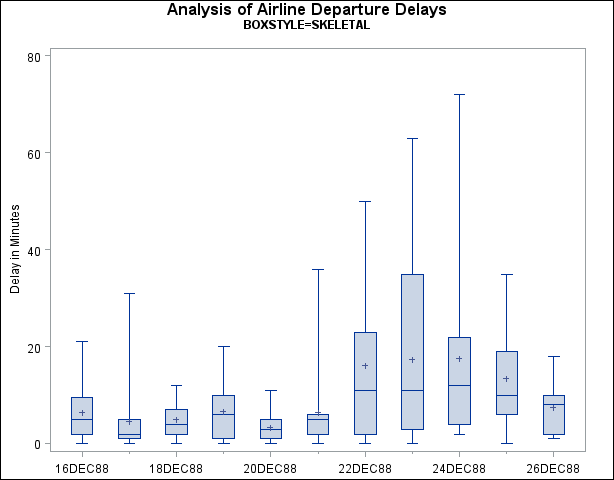The following statements request a schematic box:

```title 'Analysis of Airline Departure Delays';
title2 'BOXSTYLE=SCHEMATIC';
proc boxplot data=Times;
plot Delay*Day /
boxstyle = schematic
nohlabel;
label Delay = 'Delay in Minutes';
run;
```

When you specify BOXSTYLE=SCHEMATIC, the whiskers are drawn to the most extreme points in the group that lie within the fences. The upper fence is defined as the third quartile (represented by the upper edge of the box) plus 1.5 times the interquartile range (IQR). The lower fence is defined as the first quartile (represented by the lower edge of the box) minus 1.5 times the interquartile range. Observations outside the fences are identified with a special symbol. The default symbol is a square, and you can specify the shape and color for this symbol with the IDSYMBOL= and IDCOLOR= options. Serifs are added to the whiskers by default. For further details, see the entry for the BOXSTYLE= option. The plot is shown in Output 25.3.2.

Output 25.3.2 BOXSTYLE=SCHEMATIC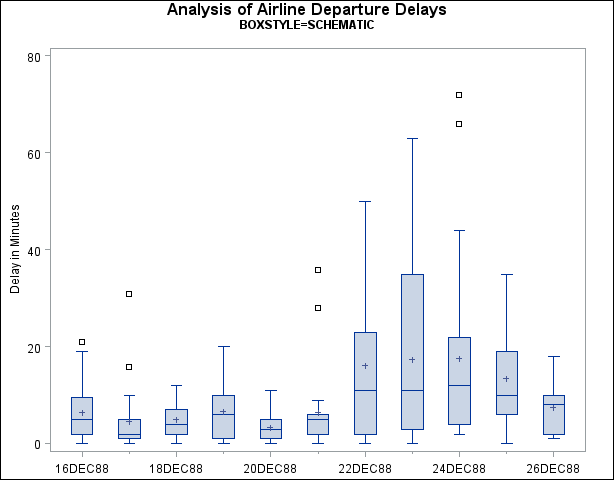The following statements create a schematic box plot in which the observations outside the fences are labeled:

```title 'Analysis of Airline Departure Delays';
title2 'BOXSTYLE=SCHEMATICID';
proc boxplot data=Times;
plot Delay*Day /
boxstyle = schematicid
nohlabel;
id Reason;
label Delay = 'Delay in Minutes';
run;
```

If you specify BOXSTYLE=SCHEMATICID, schematic box-and-whiskers plots are created and the value of the first ID variable (in this case, Reason) is used to label each observation outside the fences. The box plot is shown in Output 25.3.3.

Output 25.3.3 BOXSTYLE=SCHEMATICID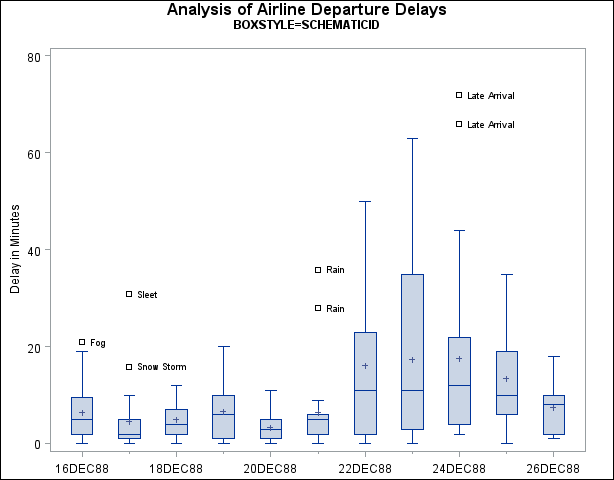The following statements create a box plot with schematic box-and-whiskers plots in which only the extreme observations outside the fences are labeled:

```title 'Analysis of Airline Departure Delays';
title2 'BOXSTYLE=SCHEMATICIDFAR';
proc boxplot data=Times;
plot Delay*Day /
boxstyle = schematicidfar
nohlabel;
id Reason;
label Delay = 'Delay in Minutes';
run;
```

If you specify BOXSTYLE=SCHEMATICIDFAR, the value of the first ID variable is used to label each observation outside the lower and upper far fences. The lower and upper far fences are located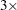IQR below the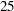th percentile andIQR above the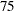th percentile, respectively. Observations between the fences and the far fences are identified with a symbol but are not labeled. The box plot is shown in Output 25.3.4.

Output 25.3.4 BOXSTYLE=SCHEMATICIDFAR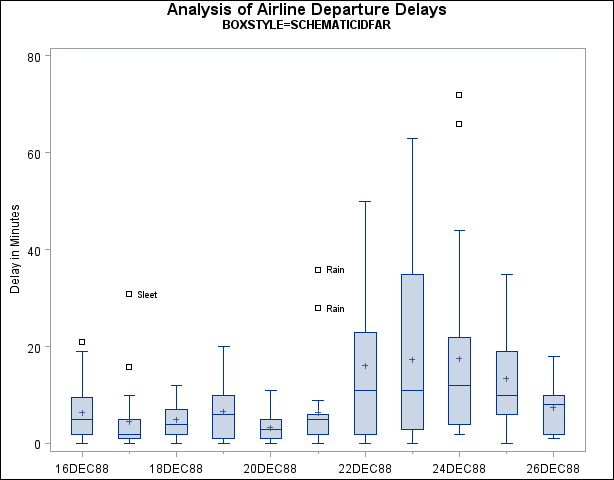Other options for controlling the display of high-resolution graphics box plots include the BOXWIDTH=, BOXWIDTHSCALE=, CBOXES=, CBOXFILL=, and LBOXES= options.